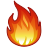# Search the Community

Showing results for tags 'dot'.

• ### Search By Tags

Type tags separated by commas.

### Forums

• General
• Lounge/General chat
• Education
• Jobs
• Marketplace
• Houdini
• General Houdini Questions
• Effects
• Modeling
• Animation & Rigging
• Lighting & Rendering
• Compositing
• Games
• Tools (HDA's etc.)
• Coders Corner
• HDK : Houdini Development Kit
• Scripting
• Art and Challenges
• Finished Work
• Work in Progress
• VFX Challenge
• Effects Challenge Archive
• Systems and Other Applications
• Other 3d Packages
• Operating Systems
• Hardware
• Pipeline
• od|force
• Feedback, Suggestions, Bugs

• 0 Replies

• 0 Views

### Interests

Found 4 results

1.## Peter Claes - Youtube channel

Hi all, As some of you may know I have recently started a youtube channel where I am sharing some techniques, setups and tips. My goal is to first cover some of the fundamental tools/setups and then build more elaborate setups. I wanted to share this with the odforce community too as this community will always have a special place in my heart :). Thank you for watching & have fun learning! The channel: https://www.youtube.com/channel/UCZMPkkgnAFghvffxaTh6CsA My first video:
• 5 replies
• 13
••2. Hello! I'm trying to kill points inside DOPs (well a popnet actually). The way I'd like to do this is by finding the closest point to the ground object (how do I reference an object in DOPs?) and computing the vector from the POP point to the nearest point, then dotting that onto the ground points normal vector. That's a nice way to figure out which side of the ground the point is on, then killing it. At least that works outside of DOPs. I'm wondering how I can do this inside DOPs because I don't want to post-process kill thousands of points if I can kill them in the simulation. Any ideas? Thanks, cw
3. http://www.hossamfx.org/math-in-houdini/ Description: This training demonstrates the big role of Math in creating visual effects and controlling the simulation behavior in Houdini FX animation software. I will cover many of the most popular math topics in computer graphics and I will show you how to apply these concepts inside Houdini with some cool projects. I will focus on what we need to know from math for using in VFX without diving into complex mathematical details as this training is not about pure math. I will show you how the Dot product can be used with the Flip Fluid, and how to create windy curves using the Cross product, and how the Matrices can determine the velocity and store the rotation data.....and many other features of using Math in Houdini. Lesson plan 1. Introduction to vectors – What is the vectors and, why we need it in math and physic and CG ?. 2. Dot product definition – Introduction to Dot product equation in math: input, return, formula, spatial situation, deficiency of Dot product . 3. Dot product and angle calculate – Simple example in Houdini for using Dot product to calculate angle between two geometry. 4. Dot product inside flip fluid 01 – Create custom force attribute for controlling fluid behavior. 5. Dot product inside flip fluid 02 – Solving deficiency in Dot product and final behavior test. 6. Cross Product definition – Introduction to Cross product equation: what we need to know about Cross Product for using in CG, and what we don't need ?. 7. Cross Product and particles 01 – Aiming dynamic force in particles by Cross product for creating windy curves (like frozen animation film). 8. Cross Product and particles 02 – Adding randomizing vector to Cross product and update vector every 10 frames. 9. Cross Product and particles 03 – Add amplitude to Cross Product direction vector and final simulation. 10. Cross Product and particles 04 – Reorient all curves by one direction using For each SOP. 11. Cross Product and particles 05 – Emitting particles from curves and creating advanced time manipulating expressions with time shift node . 12. Matrix 01 – Rotate the attributes by Matrix to save the orientation after rotation. 13. Matrix 02 – Generate Matrix using Align Vex Node and create attributes to store reorientation Matrix in, Create advanced reorient system using Matrices and For Each. 14. Matrix 03 – Without Matrix it will need a code of 20 lines to do this effect, Modify particles velocity vector by rotation matrix. 15. Sine Function 01 – What is the sine function parts in math. 16. Sine Function 02 – Adding two sine wave to simple grid. 17. Decay Solver 01 – What is the decay and what are the types of decay in life. 18. Decay Solver 02 – Creating decay solver simulation in Houdini to decay physical attributes like fluid's temperature, viscosity and cloth's wetness….etc. 19. Manipulate Rang[0-1] – Creating 10 different complex shapes from simple point number expression. ِAll Content With Project Files .
4.## math Math in Houdini FX for TDs _commercial training

hello everyone: more info here : http://www.hossamfx.org/math-in-houdini/ This training demonstrates the big role of Math in creating visual effects and controlling the simulation behavior in Houdini FX animation software. I will cover many of the most popular math topics in computer graphics and I will show you how to apply these concepts inside Houdini with some cool projects. I will focus on what we need to know from math for using in VFX without diving into complex mathematical details as this training is not about pure math. I will show you how the Dot product can be used with the Flip Fluid, and how to create windy curves using the Cross product, and how the Matrices can determine the velocity and store the rotation data.....and many other features of using Math in Houdini.
×
• Donations